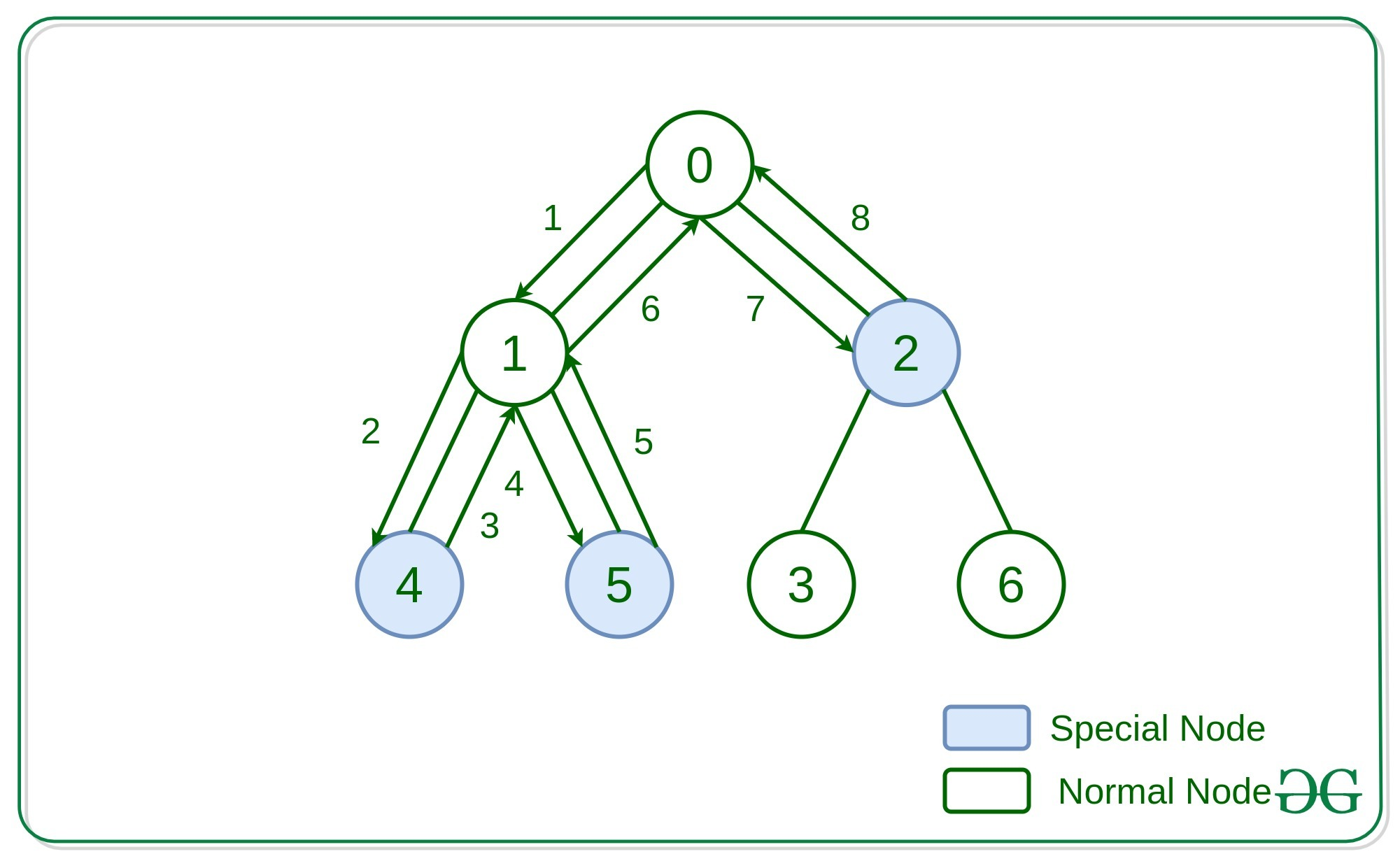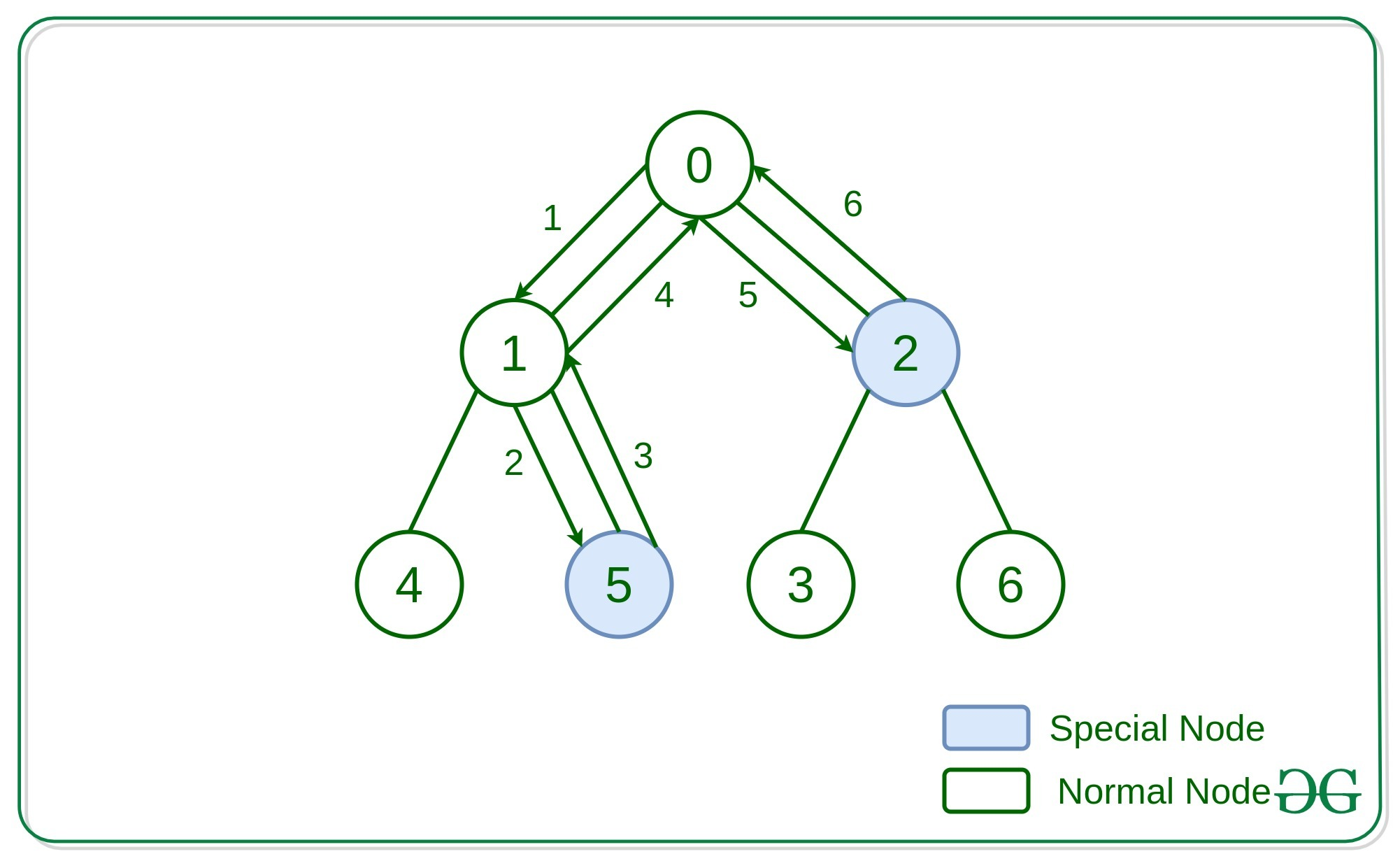# Minimum time required to visit all the special nodes of a Tree

Given an undirected tree consisting of N vertices where some of the nodes are special nodes, the task is to visit all the special nodes from the root node in minimum time. Time for travelling from one node to another node can be assumed as unit time.

A node is special if the path from the root to the node consists of distinct value nodes.

Input: N = 7, edges[] = {(0, 1), (0, 2), (1, 4), (1, 5), (2, 3), (2, 6)}
isSpecial[] = {false, false, true, false, true, true, false}
Output: 8
Explanation:Input: N = 7, edges[] = {(0, 1), (0, 2), (1, 4), (1, 5), (2, 3), (2, 6)}
isSpecial[] = {false, false, true, false, false, true, false}
Output: 6
Explanation:Approach: The idea is to use Depth First Search traversal and traverse the nodes. If any node is having a children which is a special node, add two to the steps required for that node. Also mark that node as a special node such that while moving up the steps are taken into consideration.

Below is the implementation of the above approach:

## C++

 `// C++ implementation to find ` `// the minimum time required to ` `// visit special nodes of a tree ` ` `  `#include ` `using` `namespace` `std; ` ` `  `const` `int` `N = 100005; ` ` `  `// Time required to collect ` `vector<``int``> ans(N, 0); ` ` `  `vector<``int``> flag(N, 0); ` ` `  `// Minimum time required to reach ` `// all the special nodes of tree ` `void` `minimumTime(``int` `u, ``int` `par, ` `                 ``vector<``bool``>& hasApple, ` `                 ``vector<``int``> adj[]) ` `{ ` ` `  `    ``// Condition to check if ` `    ``// the vertex has apple ` `    ``if` `(hasApple[u] == ``true``) ` `        ``flag[u] = 1; ` ` `  `    ``// Iterate all the ` `    ``// adjacent of vertex u. ` `    ``for` `(``auto` `it : adj[u]) { ` ` `  `        ``// if adjacent vertex ` `        ``// is it's parent ` `        ``if` `(it != par) { ` `            ``minimumTime(it, u, hasApple, adj); ` ` `  `            ``// if any vertex of subtree ` `            ``// it contain apple ` `            ``if` `(flag[it] > 0) ` `                ``ans[u] += (ans[it] + 2); ` ` `  `            ``// flagbit for node u ` `            ``// would be on if any vertex ` `            ``// in it's subtree contain apple ` `            ``flag[u] |= flag[it]; ` `        ``} ` `    ``} ` `} ` ` `  `// Driver Code ` `int` `main() ` `{ ` `    ``// Number of the vertex. ` `    ``int` `n = 7; ` ` `  `    ``vector<``bool``> hasApple{ ``false``, ``false``, ` `                           ``true``, ``false``, ` `                           ``true``, ``true``, ` `                           ``false` `}; ` ` `  `    ``// Store all the edges, ` `    ``// any edge represented ` `    ``// by pair of vertex ` `    ``vector > edges; ` ` `  `    ``// Added all the ` `    ``// edge in edges vector. ` `    ``edges.push_back(make_pair(0, 1)); ` `    ``edges.push_back(make_pair(0, 2)); ` `    ``edges.push_back(make_pair(1, 4)); ` `    ``edges.push_back(make_pair(1, 5)); ` `    ``edges.push_back(make_pair(2, 3)); ` `    ``edges.push_back(make_pair(2, 6)); ` ` `  `    ``// Adjacent list ` `    ``vector<``int``> adj[n]; ` ` `  `    ``for` `(``int` `i = 0; i < edges.size(); i++) { ` `        ``int` `source_node = edges[i].first; ` ` `  `        ``int` `destination_node ` `            ``= edges[i].second; ` ` `  `        ``adj[source_node] ` `            ``.push_back(destination_node); ` ` `  `        ``adj[destination_node] ` `            ``.push_back(source_node); ` `    ``} ` ` `  `    ``// Function Call ` `    ``minimumTime(0, -1, hasApple, adj); ` ` `  `    ``cout << ans; ` `    ``return` `0; ` `} `

Output:

```8
```

Attention reader! Don’t stop learning now. Get hold of all the important DSA concepts with the DSA Self Paced Course at a student-friendly price and become industry ready.

My Personal Notes arrow_drop_upCheck out this Author's contributed articles.

If you like GeeksforGeeks and would like to contribute, you can also write an article using contribute.geeksforgeeks.org or mail your article to contribute@geeksforgeeks.org. See your article appearing on the GeeksforGeeks main page and help other Geeks.

Please Improve this article if you find anything incorrect by clicking on the "Improve Article" button below.

Article Tags :
Practice Tags :

2

Please write to us at contribute@geeksforgeeks.org to report any issue with the above content.